# RD Sharma Solutions - Chapter 19 - Visualising Shapes (Part - 1), Class 8, Maths Notes | Study RD Sharma Solutions for Class 8 Mathematics - Class 8

## Class 8: RD Sharma Solutions - Chapter 19 - Visualising Shapes (Part - 1), Class 8, Maths Notes | Study RD Sharma Solutions for Class 8 Mathematics - Class 8

The document RD Sharma Solutions - Chapter 19 - Visualising Shapes (Part - 1), Class 8, Maths Notes | Study RD Sharma Solutions for Class 8 Mathematics - Class 8 is a part of the Class 8 Course RD Sharma Solutions for Class 8 Mathematics.
All you need of Class 8 at this link: Class 8

PAGE NO 19.9:

Question 1:

What is the least number of planes that can enclose a solid? What is the name of the solid?

The least number of planes that can enclose a solid is 4.Tetrahedron is a solid with four planes (faces).

Question 2:

Can a polyhedron have for its faces:
(i) 3 triangles?
(ii) 4 triangles?
(iii) a square and four triangles?

(i)No, because in order to complete a polyhedron, we need at least four triangular faces.

(ii)Yes, a polyhedron with 4 triangular faces is a tetrahedron.

(iii)Yes, with the help of a square bottom and four triangle faces, we can form a pyramid.

Question 3:

Is it possible to have a polyhedron with any given number of faces?

Yes, it is possible to have a polyhedron with any number of faces.

The only condition is that there should be at least four faces.

This is because there is no possible polyhedron with 3 or less faces.

Question 4:

Is a square prism same as a cube?

Yes, a square prism and a cube are the same.Both of them have 6 faces, 8 vertices and 12 edges.

The only difference is that a cube has 6 equal faces, while a square prism has a shape like a cuboid with two squarefaces, one at the top and the other at the bottom and with, possibly, 4 rectangular faces in between.

Question 5:

Can a polyhedron have 10 faces, 20 edges and 15 vertices?

No, because every polyhedron satisfies Euler's formula, given below: F + V = E + 2

Here, number of faces F  =  10

Number of edges E  =  20

Number of vertices V  =  15

So, by Euler's formula:

LHS: 10 + 15  =  25

RHS:  20 + 2  =  22,

which is not true because 25≠22

Hence, Eulers formula is not satisfied and no polyhedron may be formed.

Question 6:

Verify Euler's formula for each of the following polyhedrons: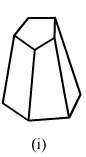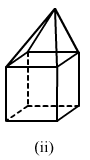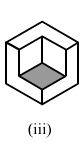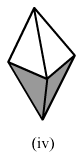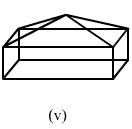(i)In the given polyhedron:Edges E = 15

Faces F = 7

Vertices V = 10

(i)In the given polyhedron:Edges E = 15

Faces F = 7

Vertices V = 10Now, putting these values in Euler's formula:

LHS: F + V =  7 + 10 =  17

LHS: E + 2 = 15 + 2 = 17

LHS  =  RHS

Hence, the Euler's formula is satisfied.Now, putting these values in Euler's formula:

LHS: F + V =  7 + 10 =  17

LHS: E + 2 = 15 + 2 = 17

LHS  =  RHS

Hence, the Euler's formula is satisfied.

(ii)In the given polyhedron:Edges E = 16

Faces F = 9

Vertices V = 9

(ii)In the given polyhedron:Edges E = 16

Faces F = 9

Vertices V = 9Now, putting these values in Euler's formula:

RHS: F + V =  9 + 9 =  18

LHS: E + 2 = 16 + 2 = 18

LHS  =  RHS

Hence, Euler's formula is satisfied.

Now, putting these values in Euler's formula:

RHS: F + V =  9 + 9 =  18

LHS: E + 2 = 16 + 2 = 18

LHS  =  RHS

Hence, Euler's formula is satisfied.

(iii)In the following polyhedron:Edges E = 21

Faces F = 9

Vertices V = 14

(iii)In the following polyhedron:Edges E = 21

Faces F = 9

Vertices V = 14Now, putting these values in Euler's formula:

LHS: F + V =  9 + 14 =  23

RHS: E + 2 = 21 + 2 = 23

This is true.

Hence, Euler's formula is satisfied.Now, putting these values in Euler's formula:

LHS: F + V =  9 + 14 =  23

RHS: E + 2 = 21 + 2 = 23

This is true.

Hence,

Euler's formula is satisfied.

(iv)In the following polyhedron:Edges E = 8

Faces F = 5

Vertices V = 5

(iv)In the following polyhedron:Edges E = 8

Faces F = 5

Vertices V = 5Now, putting these values in Euler's formula:

LHS: F + V =  5 + 5 =  10

RHS: E + 2 = 8 + 2 = 10

LHS  =  RHS

Hence, Euler's formula is satisfied.Now, putting these values in Euler's formula:

LHS: F + V =  5 + 5 =  10

RHS: E + 2 = 8 + 2 = 10

LHS  =  RHS

Hence, Euler's formula is satisfied.

(v)In the following polyhedron:

Edges E = 16

Faces F = 9

Vertices V = 9(v)

In the following polyhedron:Edges E = 16

Faces F = 9

Vertices V = 9Now, putting these values in Euler's formula:

LHS: F + V =  9 + 9 =  18

RHS: E + 2 = 16 + 2 = 18

LHS  =  RHS

Hence, Euler's formula is satisfied.

PAGE NO 19.10:

Question 7:

Using Euler's formula find the unknown:

 Faces ? 5 20 Vertices 6 ? 12 Edges 12 9 ?

We know that the Euler's formula is: F + V  =  E + 2

(i) The number of vertices V is 6 and the number of edges E is 12.

Using Euler's formula:

F + 6  =  12 + 2

F + 6 =  14

F  =  14 - 6

F  =  8

So, the number of faces in this polyhedron is 8.

(ii)Faces, F  =  5

Edges, E  =  9.

We have to find the number of vertices.

Putting these values in Euler's formula:

5 + V  =  9 + 25 + V  =  11

V  =  11 - 5

V  =  6

So, the number of vertices in this polyhedron is 6.

(iii)Number of faces F  =  20

Number of vertices V  =  12

Using Euler's formula:

20 + 12  =   E + 2

32  =  E + 2

E + 2  =  32

E  =  32 - 2

E  =  30.

So, the number of edges in this polyhedron is 30.

The document RD Sharma Solutions - Chapter 19 - Visualising Shapes (Part - 1), Class 8, Maths Notes | Study RD Sharma Solutions for Class 8 Mathematics - Class 8 is a part of the Class 8 Course RD Sharma Solutions for Class 8 Mathematics.
All you need of Class 8 at this link: Class 8Use Code STAYHOME200 and get INR 200 additional OFF

## RD Sharma Solutions for Class 8 Mathematics

88 docs

Track your progress, build streaks, highlight & save important lessons and more!

,

,

,

,

,

,

,

,

,

,

,

,

,

,

,

,

,

,

,

,

,

,

,

,

,

,

,

;